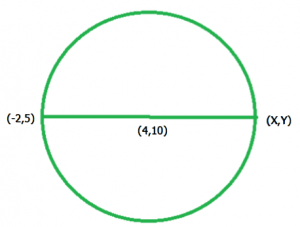# Find the other-end coordinates of diameter in a circle

Given Center coordinate (c1, c2) and one coordinate (x1, y1) of the diameter of a circle, find the other end coordinate point (x2, y2) of diameter.Examples:

Input  : x1 = –1, y1 = 2, and c1 = 3, c2 = –6
Output : x2 = 7, y2 = -14

Input  : x1 = 6.4, y1 = 3 and c1 = –10.7, c2 = 4
Output : x2 = -27.8, y2 = 5


## Recommended: Please try your approach on {IDE} first, before moving on to the solution.

The Midpoint Formula:
The midpoint of two ends coordinates points, (x1, y2) and (x2, y2) is the point M can be found by using:We have need of a (x2, y2) cordinates so we apply the midpoint the formula

          c1 = ((x1+x2)/2),  c2 = ((y1+y2)/2)
2*c1 = (x1+x2),  2*c2 = (y1+y2)
x2 = (2*c1 - x1),  y2 = (2*c2 - y1)


## C++

 // CPP program to find the   // other-end point of diameter  #include  using namespace std;     // function to find the  // other-end point of diameter  void endPointOfDiameterofCircle(int x1,                      int y1, int c1, int c2)  {      // find end point for x cordinates      cout << "x2 = "              << (float)(2 * c1 - x1)<< "  ";             // find end point for y cordinates      cout << "y2 = " << (float)(2 * c2 - y1);         }  // Driven Program  int main()  {      int x1 = -4, y1 = -1;      int c1 = 3, c2 = 5;             endPointOfDiameterofCircle(x1, y1, c1, c2);             return 0;  }

## Java

 // Java program to find the other-end point of   // diameter  import java.io.*;     class GFG {             // function to find the other-end point of       // diameter      static void endPointOfDiameterofCircle(int x1,                          int y1, int c1, int c2)      {                     // find end point for x cordinates          System.out.print( "x2 = "                             + (2 * c1 - x1) + " ");                     // find end point for y cordinates          System.out.print("y2 = " + (2 * c2 - y1));      }             // Driven Program      public static void main (String[] args)      {          int x1 = -4, y1 = -1;          int c1 = 3, c2 = 5;                     endPointOfDiameterofCircle(x1, y1, c1, c2);      }  }     // This code is contributed by anuj_67.

## Python 3

 # Python3 program to find the   # other-end point of diameter     # function to find the  # other-end point of diameter  def endPointOfDiameterofCircle(x1, y1, c1, c2):         # find end point for x cordinates      print("x2 =", (2 * c1 - x1), end=" ")             # find end point for y cordinates      print("y2 =" , (2 * c2 - y1))         # Driven Program  x1 = -4 y1 = -1 c1 = 3 c2 = 5        endPointOfDiameterofCircle(x1, y1, c1, c2)         # This code is contributed by Smitha.

## C#

 // C# program to find the other -   // end point of diameter  using System;  class GFG {             // function to find the other - end      // point of  diameter      static void endPointOfDiameterofCircle(int x1,                                             int y1,                                             int c1,                                              int c2)      {          // find end point for x cordinates          Console.Write("x2 = "+ (2 * c1 - x1) + " ");                     // find end point for y cordinates          Console.Write("y2 = " + (2 * c2 - y1));      }             // Driver Code      public static void Main ()      {          int x1 = -4, y1 = -1;          int c1 = 3, c2 = 5;                     endPointOfDiameterofCircle(x1, y1, c1, c2);      }  }     // This code is contributed by anuj_67.

## PHP

 

Output

x2 = 10 y2 = 11


Similarly if we given a center (c1, c2) and other end codrdinate (x2, y2) of a diameter and we finding a (x1, y1) cordinates

 Proof for (x1, y1) :
c1 = ((x1+x2)/2),  c2 = ((y1+y2)/2)
2*c1 = (x1+x2),  2*c2 = (y1+y2)
x1 = (2*c1 - x2),  y1 = (2*c2 - y2)


So The other end coordinates (x1, y1) of a diameter is

         x1 = (2*c1 - x2),  y1 = (2*c2 - y2)


My Personal Notes arrow_drop_upCheck out this Author's contributed articles.

If you like GeeksforGeeks and would like to contribute, you can also write an article using contribute.geeksforgeeks.org or mail your article to contribute@geeksforgeeks.org. See your article appearing on the GeeksforGeeks main page and help other Geeks.

Please Improve this article if you find anything incorrect by clicking on the "Improve Article" button below.

Improved By : vt_m, Smitha Dinesh Semwal